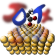JDFTx  1.5.0dump

## Syntax:

dump <freq> <var> <var> ...


## Description:

<freq> is one of:

• Electronic: Dump specified vars every (few) electronic step(s)
• End : Dump specified vars at the end of the calculation
• Fluid : Dump specified vars every (few) fluid step(s)
• Gummel : Dump specified vars every (few) fluid+electron minimize of the gummel loop
• Init : Dump specified vars from CoreDensity|Gvectors|IonicPositions|Kpoints|Lattice|None|Symmetries|Vlocps after initialization (even in dry run)
• Ionic : Dump specified vars every (few) ionic / lattice step(s)

and each <var> is one of:

• BandEigs : Band Eigenvalues
• BandUnfold : Unfold band structure from supercell to unit cell (see command band-unfold)
• BGW : G-space wavefunctions, density and potential for Berkeley GW (requires HDF5 support)
• BoundCharge : Bound charge in the fluid
• BulkEpsilon : Dielectric constant of a periodic solid (see command bulk-epsilon)
• ChargedDefect : Calculate energy correction for charged defect (see command charged-defect)
• CoreDensity : Total core electron density (from partial core corrections)
• Dfluid : Electrostatic potential due to fluid alone
• Dipole : Dipole moment of explicit charges (ionic and electronic)
• DOS : Density of States (see command density-of-states)
• Dtot : Total electrostatic potential
• Dvac : Electrostatic potential due to explicit system alone
• Ecomponents : Components of the energy
• EigStats : Band eigenvalue statistics: HOMO, LUMO, min, max and Fermi level
• ElecDensity : Electronic densities (n or nup,ndn)
• ElecDensityAccum : Electronic densities (n or nup,ndn) accumulated over MD trajectory
• EresolvedDensity : Electron density from bands within specified energy ranges
• ExcCompare : Energies for other exchange-correlation functionals (see command elec-ex-corr-compare)
• Excitations : Dumps dipole moments and transition strength (electric-dipole) of excitations
• FermiDensity : Electron density from fermi-derivative at specified energy
• Fillings : Fillings
• FluidDebug : Fluid specific debug output if any
• FluidDensity : Fluid densities (NO,NH,nWater for explicit fluids, cavity function for PCMs)
• Forces : Forces on the ions in the coordinate system selected by command forces-output-coords
• Gvectors : List of G vectors in reciprocal lattice basis, for each k-point
• IonicDensity : Nuclear charge density (with gaussians)
• IonicPositions : Ionic positions in the same format (and coordinate system) as the input file
• KEdensity : Kinetic energy density of the valence electrons
• Kpoints : List of reduced k-points in calculation, and mapping to the unreduced k-point mesh
• Lattice : Lattice vectors in the same format as the input file
• Momenta : Momentum matrix elements in a binary file (indices outer to inner: state, cartesian direction, band1, band2)
• None : Dump nothing
• Ocean : Wave functions for Ocean code
• OrbitalDep : Custom output from orbital-dependent functionals (eg. quasi-particle energies, discontinuity potential)
• QMC : Blip'd orbitals and potential for CASINO 
• RealSpaceWfns : Real-space wavefunctions (one column per file)
• RhoAtom : Atomic-orbital projected density matrices (only for species with +U enabled)
• SelfInteractionCorrection: Calculates Perdew-Zunger self-interaction corrected Kohn-Sham eigenvalues
• SlabEpsilon : Local dielectric function of a slab (see command slab-epsilon)
• SolvationRadii : Effective solvation radii based on fluid bound charge distribution
• Spin : Spin matrix elements from non-collinear calculations in a binary file (indices outer to inner: state, cartesian direction, band1, band2)
• State : All variables needed to restart calculation: wavefunction and fluid state/fillings if any
• Stress : Dumps dE/dR_ij where R_ij is the i'th component of the j'th lattice vector
• Symmetries : List of symmetry matrices (in covariant lattice coordinates)
• Vcavity : Fluid cavitation potential on the electron density that determines the cavity
• VfluidTot : Total contribution of fluid to the electron potential
• Vlocps : Local part of pseudopotentials
• Vscloc : Self-consistent potential
• XCanalysis : Debug VW KE density, single-particle-ness and spin-polarzied Hartree potential

List of dumped variables from multiple instances will be accumulated for each <freq>. Use command dump-interval to dump at regular intervals instead of every iteration.

## Properties:

Requires:     (None)

Forbids:     (None)

Allow multiple:    yes

Default:

dump End State